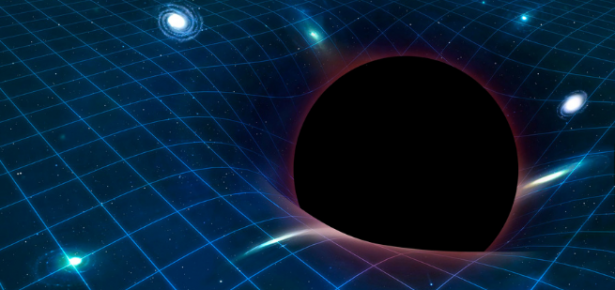Products and services

# Fifteen Eighty Four

29
Apr
2021

## What is Gravity?

Kirill KrasnovAlbert Einstein’s crown achievement was the realisation that one can/should equate:

Gravity=Geometry

At the time Einstein was inventing his dynamical theory of geometry there was only one “geometry” that could possibly appear on the right-hand side of this equation. This is the geometry of Carl Friedrich Gauss and Bernhard Riemann as became known to Einstein via his mathematician friend Marcel Grossmann

Geometry now is a much richer subject than it was at the beginning of 20th century, with revolutionary contributions from Elie Cartan in particular. One of Cartan’s main achievements was the realisation that a metric is just one possible geometric structure, and that one can define geometric structures of other types. We now understand that apart from Riemannian geometry of metrics there is also symplectic, complex, Kaehler, quaternionic etc. geometries, all determined by the geometric structure that is used as the “model”. All this also relates to the beautiful subject of the theory of Lie groups, the second of Cartan’s main achievements.

It is then interesting and natural to ask how much of the statement “Gravity=Geometry” depends on the particular type of geometry used. The monograph “Formulations of General Relativity” explores precisely this question and shows that gravity can be equally well described using geometries different from the metric geometry that forms the basis of Einstein’s original theory of General Relativity. Cartan’s differential geometry of “moving frames” is explained and the corresponding formalism for gravity is developed. However, the monograph pays a special attention to General Relativity in four spacetime dimensions. As is explained in the book, there is a special algebraic phenomenon that is known to occur in this number of dimensions, which implies that there exists a series of “chiral” formulations of gravity. These chiral formalisms for 4D General Relativity are considered in detail in the book, and are based on a special type of geometry, one related to the concept of self-duality. This links the geometric considerations of relevance for gravity to such concepts as spinors and twistors.

The main message that the monograph advocates is that while gravity is undeniably about geometry, one should keep one’s options open as to what the geometry that is ultimately relevant for the description of our world is.

 Albert Einstein (1879-1955) was a German-born theoretical physicist and is most known for developing the theory of relativity.
 Carl Friedrich Gauss (1777-1855) was a German mathematician who made significant contributions to many of its fields and is generally regarded as one of the greatest mathematicians of all time.
 Bernhard Riemann (1826-1866) was a German mathematician who made pioneering contributions to analysis, number theory and differential geometry.
 Marcel Grossmann (1878-1936) was a Swiss mathematician and a friend and classmate of Albert Einstein.
 Elie Cartan (1869-1951) was an influential French mathematician who did fundamental work in the theory of Lie groups, differential systems and differential geometry.